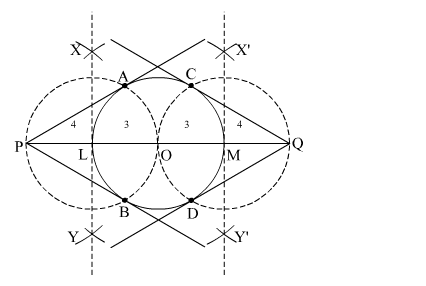# Draw a circle of radius 3 cm. Take two points P and Q on one of its diameters extended on both sides, each at a distance of 7 cm`
Question:

Draw a circle of radius 3 cm. Take two points P and on one of its diameters extended on both sides, each at a distance of 7 cm on opposite sides of its centre. Draw

tangents to the circle from these two points and Q.

Solution:

Steps of Construction

Step 1. Draw a circle with O as centre and radius 3 cm.

Step 2. Mark a point P and Q on one of its diameters extended on both sides outside the circle such that OP = OQ = 7 cm.

Step 3. Join OP and OQ. Draw the perpendicular bisector XY of OP and X'Y' of OQ, cutting OP at L and OQ at M.

Step 4. Draw a circle with L as centre and radius PL (or OL), to intersect the given circle at the points A and B. Draw another circle with M as centre and radius MQ (or OM), to intersect the given circle at the points C and D.

Step 5. Join PA and PB. Join QC and QD.Here, PA, PB and QC, QD are the required tangents.